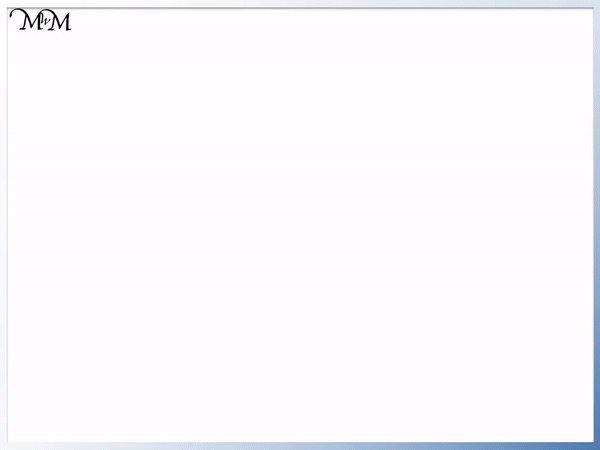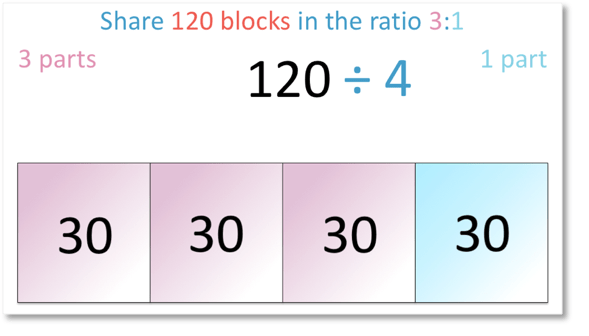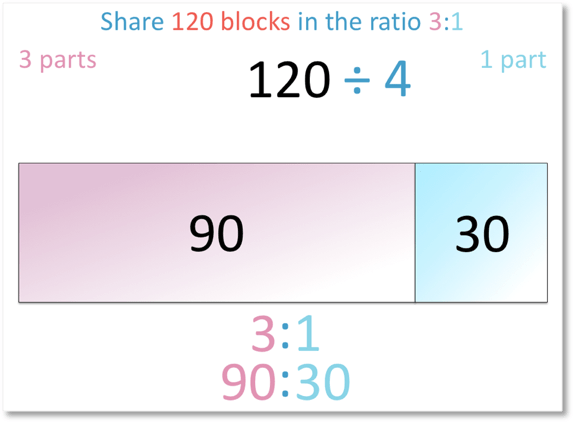# Sharing in a Ratio: Bar Model

Sharing in a Ratio: Bar Model• We can use the bar model to show our given amount as a rectangular bar.
• In this example, the whole bar is worth 120.
• We can divide the bar into the total number of parts in the ratio.
• There are 3 + 1 = 4 parts in the ratio, each worth an equal amount.
• 120 ÷ 4 = 30.
• Each part of our bar model is worth 30.
• 3 of the parts are worth 90 in total.
• The remaining part is worth 30.
• 120 shared in the ratio of 3:1 is 90:30.
The complete bar in the bar model represents the total amount and we divide it into equal parts to show the proportions in our ratio.• In this example we will share 42 grams of sugar in the ratio 3:4 using the bar model.
• The whole bar represents 42 g.
• There are 3 + 4 = 7 parts in the ratio in total.
• We divide the bar into 7 equal parts.
• 42 g ÷ 7 = 6 g.
• The 3 parts in our ratio are worth 3 x 6 g = 18 g
• The 4 parts in our ratio are worth 4 x 6 g = 24 g.
• 42 g shared in the ratio 3:4 = 18:24.Supporting Lessons# How to Share an Amount in a Given Ratio using a Bar Model

In this lesson we will learn how to share an amount in a given ratio using the bar model.

The bar model is a simple method of showing proportion visually and introducing sharing in a ratio. A rectangular bar is drawn to represent the total amount and it is divided into equal parts in accordance with the given ratio.

Here is our first ratio problem:We are asked to share 120 blocks of chocolate in the ratio 3:1.

There are two numbers in our ratio problem, ‘3’ and ‘1’. This means that we are sharing the 120 blocks between two people.

This means that for every three parts one person is given, the second person receives one part.

We will show the three parts in pink and the one part in blue on our rectangular bar model.The complete rectangle in the bar model above represents all 120 blocks of chocolate.

The bar model will be divided into equal parts.

There are 3 parts for one person and 1 part for the other person. This is four parts in total.

This means we divide the bar into 4 equal parts.Three parts of our bar will be given to one person. These are shown in pink.

One part of the bar will be given to the second person. This is shown in blue.

We need to find out how much each part is worth.We must share the 120 blocks over the four equal parts using division.

Because we want to share the blocks equally, we will divide 120 by 4.

120 ÷ 4 = 30

Therefore, each of the four parts on the bar model are worth 30 blocks.We will now look at the three pink parts to find out how many blocks of chocolate the first person will receive in total.We have three parts, each worth 30.

30 x 3 = 90

The first person therefore receives 90 blocks of chocolate.The second person receives one lot of 30.

So, they receive 30 blocks of chocolate.The chocolate has been shared in a ratio of 90:30.

When teaching ratio problems for the first time, the bar model can be an easy way to show the situation visually.Now try our lesson on Solving Ratio Problems where we learn the method for solving ratio problems.